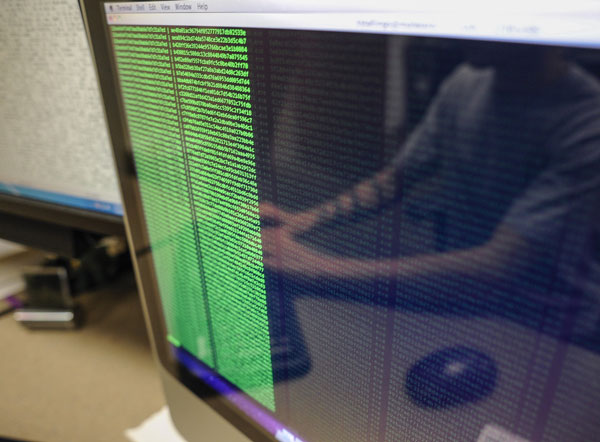M.S. in Computer Science (MSCS) Prerequisites

The following computer science and mathematics courses should be taken before applying to the MSCS program.

Computer Science RequirementsThe following UAB computer science courses, or their equivalents, are required of all applicants before they can apply to our graduate program:

• CS 103: Introduction to Computation
• CS 203: Introduction to Object-Oriented Programming
• CS 250: Discrete Structures
• CS 303: Algorithms and Data Structures

The following courses are also required but can be taken after the student is admitted, up to three of which may be taken at the 500-level and counted toward the MSCS program:

• CS 401/501: Programming Languages
• CS 420/520: Software Engineering
• CS 433/533: Operating Systems

Mathematics Requirements

Mathematics background equivalent to two terms of calculus (differential and integral calculus) is required. Linear algebra, statistics, differential equations, and advanced calculus are highly recommended.SaveSaveSaveSave